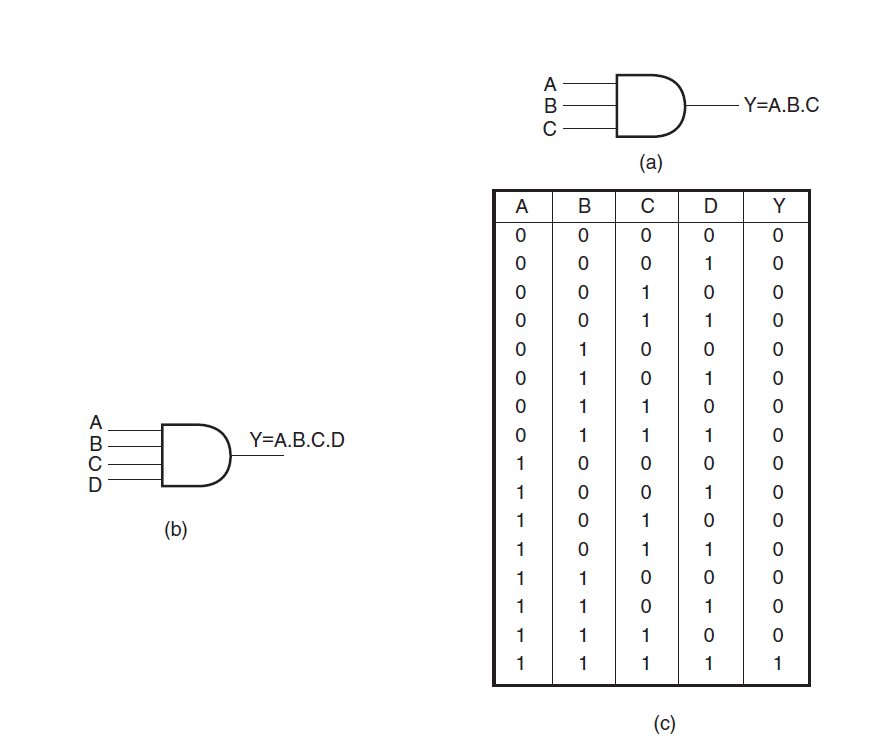# AND Gate – definition, symbol, & Truth Table

An AND gate is a logic circuit having two or more inputs and one output. The output of an AND gate is HIGH only when all of its inputs are in the HIGH state. In all other cases, the output is LOW. When interpreted for a positive logic system, this means that the output of the AND gate is a logic ‘1’ only when all of its inputs are in logic ‘1’ state. In all other cases, the output is logic ‘0’.

The logic symbol and truth table of a two-input AND gate are shown in Figs 1(a) and (b) respectively.

Figures 2(a) and (b) show the logic symbols of three-input and four-input AND gates respectively. Figure 2(c) gives the truth table of a four-input AND gate.Figures 2(a) and (b) show the logic symbols of three-input and four-input AND gates respectively. Figure 2(c) gives the truth table of a four-input AND gate.

The AND operation on two independent logic variables A and B is written as Y = A.B and reads as Y equals A AND B and not as A multiplied by B. Here, A and B are input logic variables and Y is the output.

An AND gate performs an ANDing operation:

• for a two-input AND gate, Y = A.B
• for a three-input AND gate, Y = A.B.C
• for a four-input AND gate, Y = A.B.C.D

If we interpret the basic definition of OR and AND gates for a negative logic system, we have an interesting observation. We find that an OR gate in a positive logic system is an AND gate in a negative logic system. Also, a positive AND is a negative OR.